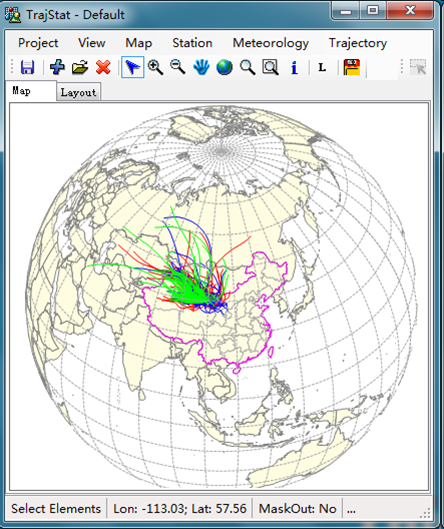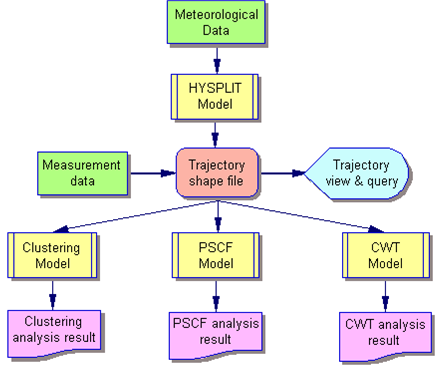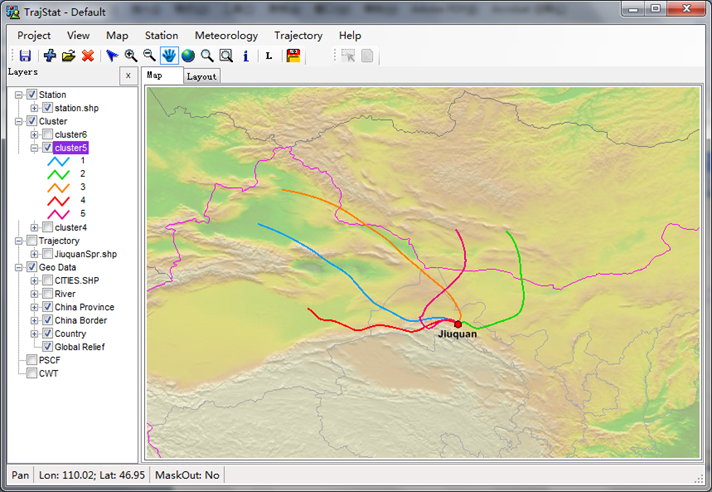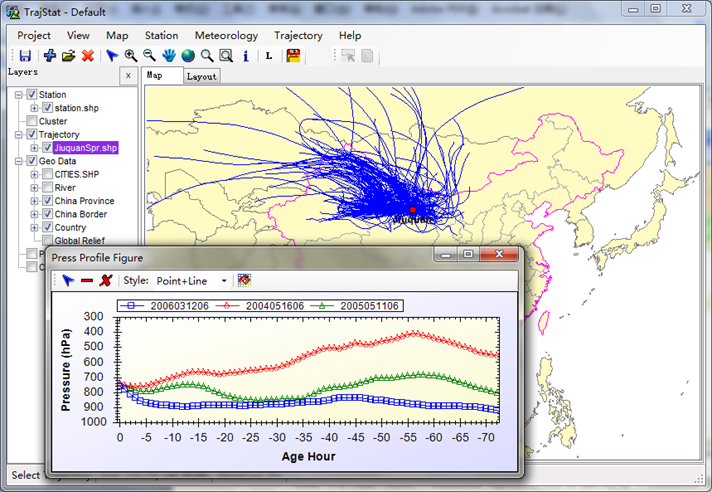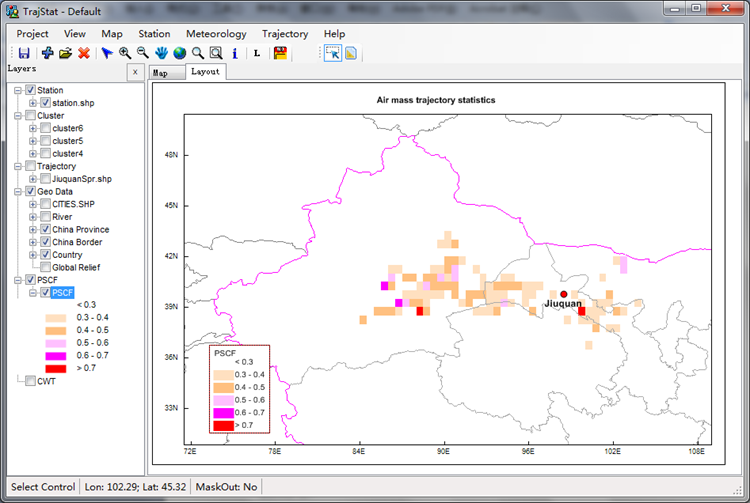# TrajStat¶

## Introduction:¶

Statistical analysis of air mass back trajectories combined with long-term ambient air pollution measurements are useful tools for source identification. Using these methods, the geographic information system (GIS) based software, TrajStat, was developed to view, query, and cluster the trajectories and compute the potential source contribution function (PSCF) and concentration weighted trajectory (CWT) analyses when measurement data are included.

The HYSPLIT model is used to calculate trajectories, which are loaded into the system as an external process. The trajectory files with three-dimensional endpoint data could be converted to the ESRI “PolylineZ” shape file format. In this type of shape file the x, y and z properties of each point are defined by its longitude, latitude and air pressure along the trajectory. The trajectories can be shown in various spatial patterns. For instance, using only the level (x, y) or height (z) coordinates, each trajectory can be shown as a two-dimensional figure. When combined height with longitude and latitude values, the three-dimensional trajectories can be plotted. The long-term measurement data could be assigned to their corresponding trajectories. A query function was developed to identify the trajectories to which a user can distinguish the polluted trajectories with high measurement concentration from a large number of trajectories and then the pollutant pathway could be roughly estimated. Euclidean distance or angle distance (Sirois and Bottenheim, 1995) can be selected as the cluster model. A reasonable maximum cluster number can be decided through visual inspection and comparison of the mean-trajectory maps. The mean pollutant concentration for each cluster can be computed using the cluster statistics function. Pollutant pathways could then be associated with the high concentration clusters. After calculating the PSCF and CWT value, an arbitrary weight function (Polissar et al., 1999) is applied to reduce the uncertainty of cells with few endpoints. Then the potential source regions with high PSCF or CWT value could be identified.

## Publication:¶

• Wang, Y.Q., Zhang, X.Y. and Draxler, R., 2009. TrajStat: GIS-based software that uses various trajectory statistical analysis methods to identify potential sources from long-term air pollution measurement data. Environmental Modelling & Software, 24: 938-939

## Source code¶

https://github.com/Yaqiang/TrajStat

## Screen shots:¶